Estimation of the Maximum Concentration for Non-Gaussian Under Using Different Schemes of Dispersion Parameters for Isotopes | OMICS International
Journal of Civil & Environmental Engineering

# Estimation of the Maximum Concentration for Non-Gaussian Under Using Different Schemes of Dispersion Parameters for Isotopes

 Essa KSM* and Elsaid SEM Mathematics and Theoretical Physics, NRC, Atomic Energy Authority, Cairo, Egypt Corresponding Author : Essa KSM Mathematics and Theoretical Physics NRC, Atomic Energy Authority, Cairo, Egypt Tel: 989378212956 E-mail: [email protected] Received: June 23, 2015 Accepted: July 08, 2015 Published: July 18, 2015 Citation: Essa KSM, Elsaid SEM (2015) Estimation of the Maximum Concentration for Non-Gaussian Under Using Different Schemes of Dispersion Parameters for Isotopes. J Civil Environ Eng 5:178. doi:10.4172/2165-784X.1000178 Copyright: © 2015 Essa KSM, et al. This is an open-access article distributed under the terms of the Creative Commons Attribution License, which permits unrestricted use, distribution, and reproduction in any medium, provided the original author and source are credited. Related article at Pubmed, Scholar Google

Visit for more related articles at Journal of Civil & Environmental Engineering

#### Abstract

In this paper, we have calculated of the maximum concentration for non-Gaussian and maximum downwind distance under using different schemes of dispersion parameters for isotopes. We have compared between maximum predicated, concentrations for non-Gaussian under using different schemes of dispersion parameters for I131 and Cs137 via observed and maximum downwind distance.

 Keywords Dispersion parameters for isotopes; Maximum downwind distance; Non-Gaussian Introduction Atmospheric dispersion modeling refers to the mathematical description of contaminant transport in the atmosphere. The term dispersion in this context used to describe the combination of diffusion (due to turbulent eddy motion) and advection (due to the wind). Analytical and approximate solutions for the atmospheric dispersion problem have been derived under wide range of simplifying assumptions, as well as various boundary conditions and parameter dependencies. These analytical solutions are especially useful to engineers and environmental scientists who study pollutant transport, since they allow parameter sensitivity and source estimation studies to be performed . Both our scientific understanding and technical developments have greatly increased by the use of empirical, analytical and numerical models to predict the air pollution concentration in atmosphere. For this purposed, the advection – diffusion equation has been largely applied in operational atmospheric dispersion models, in principal, from this equation it is possible to obtain the dispersion from a source given appropriate boundary and initial conditions plus knowledge of the mean wind velocity and concentration turbulent fluxes . The advection–diffusion equation has largely calculated in operational atmospheric dispersion models to predict mean concentrations of contaminants in the planetary boundary dispersion from a continuous point source given appropriate boundary and initial conditions as well as knowledge of the mean wind velocity and concentration turbulent fluxes. Many turbulent dispersion studies are relating to the specification of these turbulent fluxes to allow the solution of the averaged advection –diffusion equation, this procedure used to know as the closure of the turbulent diffusion problem. In this paper, we have calculated of the maximum concentration for non-Gaussian and maximum downwind distance under using different schemes of dispersion parameters for isotopes. We have compared between maximum predicated, concentrations for non -Gaussian under using different schemes of dispersion parameters for I131 and Cs137 via observed and maximum downwind distance. Non-Gaussian distributions The concentration from a continuous point source of strength Q with interference from the ground at a mean wind speed U using non- Gaussian plume formula as follows :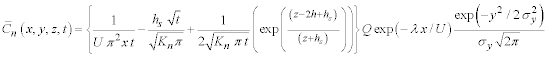(1) Where: C is the mean concentration of the effluent at a point (x, y, z), (Bq/m3). Q is the source strength (Bq). U is the mean wind speed (m/s). X, y, z refer to a downwind, crosswind and vertical coordinate system at the center of the moving cloud. Σi (i=x, y, z) are the plume dispersion coefficients in the x, y and z directions respectively (m) [4,5]. Exp(-x λ/U) is the radioactive decay for the specified nuclide. H is the effective stack height {hs (stack height) +Δ h (plume rise)} (m). Maximum mean concentration of the effluent concentration occurs when ∂Cn/∂x=0 which gives: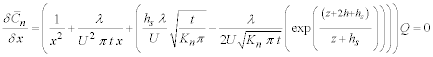(2) From which we get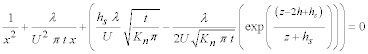(3) Multiply the equation (3) in x2, we get: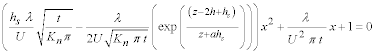(4) From which we get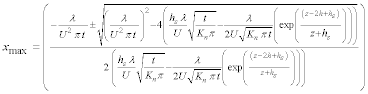(5) Substituting from equation (5) on equation (1), we get maximum mean concentration of the effluent concentration:-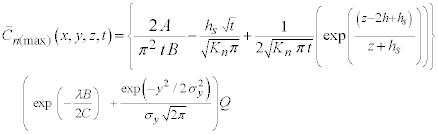(6) Where A=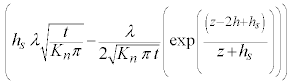B=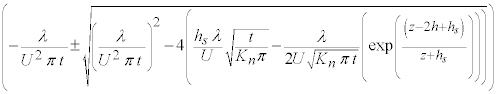C=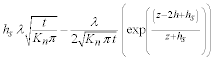Dispersion parameters schemes We select the four different methods namely, power law , Briggs, Irwin and standard method for calculating σy and σz to select the most accurate one , as follows. A-Power-law method In this method, σy and σz can be calculated from the following formula: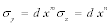Where c, d, m, n values differ according to stability classes, as given in Table 1. B- Standard method In this method, σy and σz are in the form: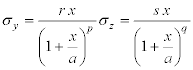Where r, s, p and q are constants depending on the atmospheric stability. These values are explained in Table 2 C-Briggs method In this method, σy and σz can be calculated from the Tables 3-6 according to Briggs . D- Irwin method In this method, σy and σz are taking the following formula: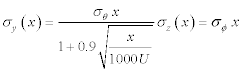Where σθ and σφ are the standard deviation of the wind direction in the horizontal and vertical directions, respectively. Specification of σθ and σφ can be found , based on the Pasquill stability classes from A to F. The comparison between observed and predicated, maximum concentrations for non-Gaussian under using different schemes of dispersion parameters for I131 are shown in Figure 1. It is clear that in case power law method the most values both predicated and maximum concentrations are near from the observed values, while the most values for both predicated and maximum concentrations are far from observed values in cases of standard, Briggs and Irwin methods. The comparison between observed and predicated, maximum concentration via maximum downwind distance for non-Gaussian under using different schemes of dispersion parameters for I131 are shown in Figure 2. It is clear that the most values of observed, predicated and maximum concentrations are far from maximum downwind distance values in cases of standard , Briggs and Irwin methods , while , the most values of observed, predicated and maximum concentrations are near from maximum downwind distance values in case power law method. The comparison between observed and predicated, maximum concentrations for non-Gaussian under using different schemes of dispersion parameters for Cs137 are shown in Figure 3. It is clear the most values of predicated and maximum concentrations agreement with observed data in cases of standard, Briggs and Irwin methods, while in case power law method the values of maximum concentration are best from predicated concentration with observed data. The comparison between observed and predicated, maximum concentration via maximum downwind distance for non-Gaussian under using different schemes of dispersion parameters for Cs137 are shown in Figure 4. It is clear in cases of Briggs and Irwin methods the values of observed and predicated concentrations are best from maximum concentrations with maximum downwind distance, while, in cases power law and standard methods the values of predicated concentration are best from observed and maximum concentrations with maximum downwind distance. Conclusion `The maximum concentration for non-Gaussian and maximum downwind distance under using different schemes of dispersion parameters for isotopes has evaluated. Comparison between maximum predicated concentrations for non-Gaussian under using different schemes of dispersion parameters for I131 and Cs137 via observed and maximum downwind distance are calculated. References Stockie JM (2011) The Mathematics of atmospheric dispersion modeling.Society for industrial and applied mathematics53: 349-372. Arya SP (1999)Air pollution meteorology and dispersion.Oxford university Press Oxford 310. Essa KSM, Elsaid SEM (2014) Time dependent advection diffusion equation in two dimensions. International Journal of Engineering and Technical Research (IJETR).Under publication. Curtiss PS, RablA (1996)Impacts of air pollution general relationships and site dependence. Atmospheric Environment 30:3331-3347. Faw RE, Shultis KJ (1993) Radiological Assessment, Sources and Exposures. PTR Prentice-Hall: Englewood Cliffs, New Jersey. Essa KSM, Fouad EA (2011) Estimating of crosswind integrated Gaussian and non-Gaussian concentration by using different dispersion schemes. Australian Journal of Basic and Applied Sciences, 5: 1580-1587. BriggsGA (1973) Diffusion estimation for small emissions. ATDL Contrib. 79 (draft), Air Resource Atmospheric Turbulence and Diffusion Laboratory, Oak Ride. Hanna SR (1989)confidence limit for air quality models as estimated by bootstrap and Jackknife resembling methods. Atom Environ 23: 1385-1395.

#### Tables and Figures at a glanceTable 1 Table 2 Table 3Table 4 Table 5 Table 6

#### Figures at a glance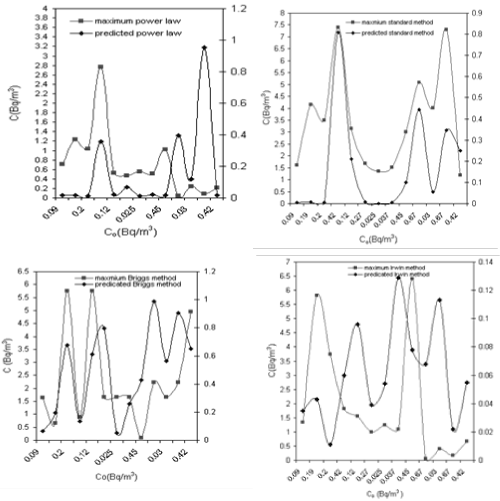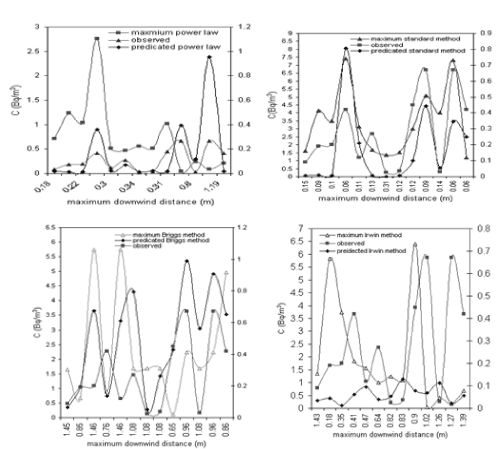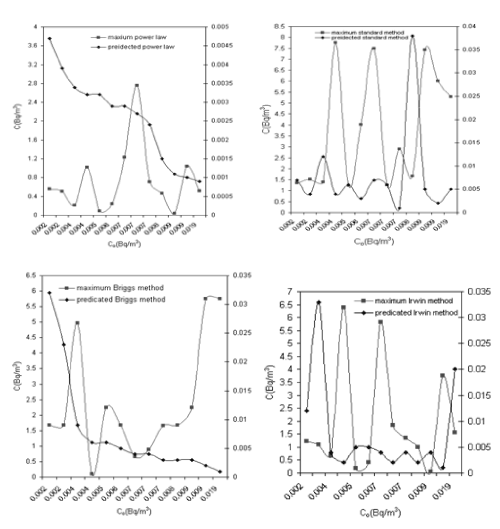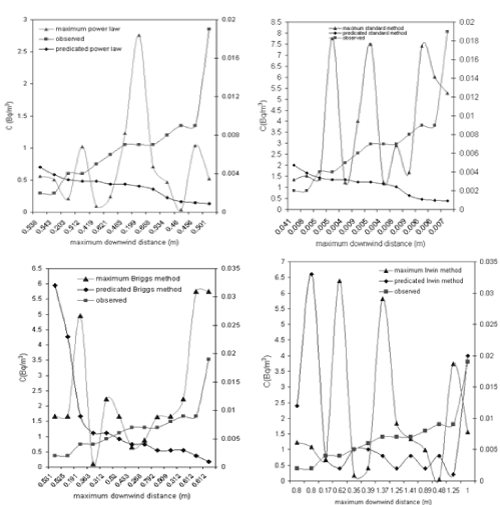Figure 1 Figure 2 Figure 3 Figure 4
Select your language of interest to view the total content in your interested language

### Article Usage

• Total views: 12161
• [From(publication date):
August-2015 - Feb 22, 2020]
• Breakdown by view type
• HTML page views : 8352Can't read the image? click here to refresh